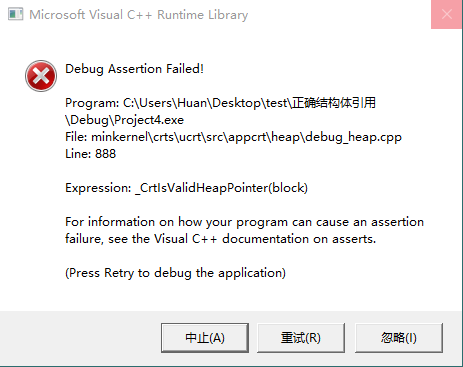`````` #define _CRT_SECURE_NO_WARNINGS
#include <iostream>
#include <iomanip>
#include <math.h>
#include <ctime>
#include <Windows.h>
using namespace std;

bool compare(const char *s, const char *q, int n)
{
int i = 0;
while (i<n)
{
if (q[i++] != s[i++])
return false;
}
if (i = n && (s[n] - ' ' == 0 || s[n] - '\n' == 0 || s[n] == NULL))
return true;
else
return false;
}

int Triangle_num(char* fname)
{
FILE *fp;
if ((fp = fopen(fname, "r ")) == NULL)
{
printf("Can't open file");
exit(1);
}

int npoint = 0; //点个数
int count = 0; //三角面片个数

while (!feof(fp))     //feof()函数为判断文件是否结束，C语言
{
char f;
fgets(f, 256, fp);
if (compare(strtok(f, "   "), "vertex ", 6))
{
if (++npoint == 3)
{
count++;
npoint = 0;
}
}
}
fclose(fp);
return   count;
}

void main()
{
char *a, *b;
a = new char;
b = new char;
a = "C:\\Users\\Huan\\Desktop\\stl\\12workpiece.STL";
b = a;
Triangle_num(a);
system("pause");

delete[] b;//增加delete之后出现报错
}
``````

2个回答

`````` char *a, *b;
a = new char;
b = new char;
strcpy(a,"C:\\Users\\Huan\\Desktop\\stl\\12workpiece.STL");

b = a;    // a 和 b 指向同一块区域
//Triangle_num(a);
//system("pause");

delete a;  释放其中之一就可以
``````

`````` #include <stdio.h>

int main(int argc, char **argv)
{
char *a, *b;
a = new char;
b = new char;

printf("a: %p b: %p\n", a, b);
a = "C:\\Users\\Huan\\Desktop\\stl\\12workpiece.STL";
b = a;
printf("a: %p b: %p\n", a, b);

return 0;
}
``````

a: 0x80010288 b: 0x800102c0
a: 0x403070 b: 0x403070

new 了 a 和 b之后，这时 a地址是0x80010288 b地址是: 0x800102c0c++ delete释放内存的问题

char* p = new char; 然后将char强转成一个结构体指针,然后在delete这个结构体指针. 当然是用delete[ ] 进行删除? 这样会有问题吗?还有就是想问delete 一个指针的时候跟这个指针类型有什么关系?

C++ 关于指针和delete的问题，delete总是报错

RT，为什么我们可以删除正在运行的程序呢？是因为程序已经被加载到内存了吗？ 可是系统不是以分页的方式将部分程序中的内容加载到内存的吗？如果需要访问 其他还没有被加载到内存的部分，可是文件已经被删除了，不会引起问题吗？ 希望好心人指教

qt异常退出： 问题描述： 第一次安装qt-windows-opensource-5.1.1-msvc2010-x86-offline.exe, 问题是构建缺少编辑器，请分配一个编辑器。 弄了许久，无法解决。于是重新安装一个 qt-windows-opensource-5.1.1-mingw48_opengl-x86-offline.exe 我什么代码都没编写，只按照qt的向导创建了一个对话框工程， 在运行的时候，就出现了 test.exe 启动中... 程序异常结束。 的错误报告！网上查了许多，有的说是因为安装两次，上一个qt卸载不干净， 在运行的时候一些动态库在运行的时候会出错,于是我重新卸载，用360清理注册表后重新安装程序，但是还是出现同样的问题。有的说是因为路径不对，需要重新 指定（备注：我在安装的时候都是按照默认的路径的）。 请各位好心人帮我看下是什么问题？

C++ delete 释放内存 不行

for(i = height*(width/1201+1);i > 0;i--) { QByteArray datagram; datagram.resize(width+11); for(j = width+12;j >= 0;j--) { datagram[j] = sendbuffer[i][j]; } sender->writeDatagram(datagram.data(),datagram.size(),QHostAddress::Broadcast,1234); delete[]senderbuffer[i]; } delete[]senderbuffer; 请问我这样释放内存为何不行？

qt的内存泄漏问题（qt中的new和delete操作）

C++程序在vs2010下运行出现错误：HEAP CORRUPTION DETECTED ........

![图片说明](https://img-ask.csdn.net/upload/201705/11/1494504897_238301.png) 我编写了一段程序，中间用到了如下分配指针和删除指针的代码。在visual sudio中，程序可以正确运行。同样的程序，在VC++6.0，也可以运行，但运行到delete的代码部分就会弹出如下报警窗口，请问这是什么原因导致的呢？ ``` for (int i = 0; i < 10; i++)//分配Tool内存 { Tool[i].file = new char; Tool[i].name = new char; for (int j = 0; j < 5000; j++) { Tool[i].p_tri[j] = new TRIANGLE; for (int k = 0; k < 3; k++) { Tool[i].p_tri[j]->p_pnt[k] = new POINT3D; } } } char *t, *n; for (int a = 0; a < 10; a++) { t[a] = Tool[a].file; n[a] = Tool[a].name; } for (int i = 0; i < 10; i++)//删除内存，如下代码在VC++6.0中运行后弹出警告窗口 { delete[] t[i]; delete[] n[i]; for (int j = 0; j < 5000; j++) { for (int k = 0; k < 3; k++) { delete Tool[i].p_tri[j]->p_pnt[k]; } delete Tool[i].p_tri[j]; } } ```

c++类的对象中分配的内存的内存能不能在在程序中间delete掉再重新分配?

Matrix& Matrix::operator=(const Matrix &mp) { if (mp.row_n != row_n||mp.column_n != column_n) { std::cout << "Error , size not match" << std::endl; return *this; } /* for (int i = 0; i < row_n; i++) { delete[] element[i]; } delete[] element; element = (int **)new int*[mp.row_n]; for (int i = 0; i < column_n; i++) element[i] = new int[mp.column_n]; */ for (int i = 0; i < row_n; i++) for (int j = 0; j < column_n; j++) element[i][j] = mp.element[i][j]; return *this; } ``` 注释中的那一句加上之后程序就报错说*** Error in `./temp': double free or corruption (out): 0x0000000000caf1d0 ***

C++内存泄露，只是动态申请对象，然后delete就发生了内存泄露，真奇怪。

java windows环境下 file.delete遇到无法删除问题，程序结束后文件随之消失

windows环境下， 调用file.delete()方法，返回true 但是文件依然可以在文件夹中看到，无法对文件进行操作 结束程序，文件消失（没有调用deleteOnExit方法） 问，，，如何直接删除文件 确认过几遍流的问题，没能在代码中找到未关闭的流

Java语言分配内存用new，那么释放内存用什么，delete不好用，还有别的技术么

Java语言分配内存用new，那么释放内存用什么，delete不好用，还有别的技术么

delete不是可以让某个指针所指向的区域按照这个指针的类型所占有的内存大小来释放空间吗，那这块区域就应该被填充了未知的垃圾数据，但是为什么被删掉的节点的数据没有被垃圾数据所覆盖呢？ ``` #include<bits/stdc++.h> using namespace std; struct node{ int number; struct node *next; }; int main(void) { struct node Node; Node.number = 1; struct node Node2; Node.next = &Node2; Node2.next = NULL; Node.number = 2; printf("%p %d\n",Node,Node.number); struct node *deletenode = &Node; delete deletenode; deletenode = NULL; printf("%p %d\n",Node,Node.number); } ``` 程序运行的结果： ![图片说明](https://img-ask.csdn.net/upload/201912/22/1576992921_411314.png) 各位大佬救救大一的孩子吧，要裂开了

C++程序运行时不能读取内存

VS++代码 char * test = new char; test='b'; delete []test; 运行delete之后出现HEAP_CORRUPTION_DETECTED错误，有哪位大神指点一二……

Java基础知识面试题（2020最新版）

Intellij IDEA 实用插件安利

1. 前言从2020 年 JVM 生态报告解读 可以看出Intellij IDEA 目前已经稳坐 Java IDE 头把交椅。而且统计得出付费用户已经超过了八成（国外统计）。IDEA 的...

MySQL数据库面试题（2020最新版）

2020阿里全球数学大赛：3万名高手、4道题、2天2夜未交卷

HashMap底层实现原理，红黑树，B+树，B树的结构原理 Spring的AOP和IOC是什么？它们常见的使用场景有哪些？Spring事务，事务的属性，传播行为，数据库隔离级别 Spring和SpringMVC，MyBatis以及SpringBoot的注解分别有哪些？SpringMVC的工作原理，SpringBoot框架的优点，MyBatis框架的优点 SpringCould组件有哪些，他们...

《Oracle Java SE编程自学与面试指南》最佳学习路线图2020年最新版（进大厂必备）